##### The Japanese Maple specialist
Direct order Contact Help / Services Newsletter# Shelf to displaySearch

Japanese MaplesYoung trees Acer seeds and more Bonsai soil and fertiliserVarieties introduced into EuropeHostasWind bells and chimesSaxifragesSpecial offer

Packing and shipping charges

# Hosta amalia

› Hostas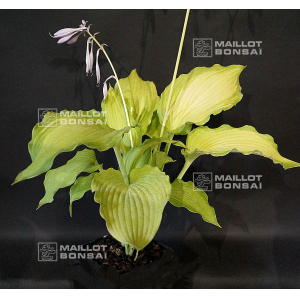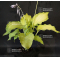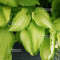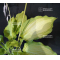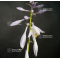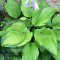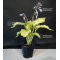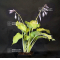ref. : 11033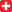12,00

Available quantity : 27Order

###### Description

Coming from a hybridization of Hosta Dancing queen.

Also called Princess Amalia.

Height : about 80 cm. Width : 60 cm.

Leaves with dark green side and green shade in the middle.

Lavender color flowers. Flowering in July/August.

Wonderful variety that is still little known in Europe but has a good future thanks to its radiant color.

Big rhizome delivered in a 1 liter plastic pot.

#amalia 3.6 #hosta 3.5 #hostas 2.6 #color 2.5 #green 2.5 #hybridization 2.3 #wonderful 1.9 #flowering 1.9 #delivered 1.9 #lavender 1.8

Formule
(( ROUND((CHAR_LENGTH(b.article_nom)-CHAR_LENGTH(REPLACE(b.article_nom, 'amalia', '')))/LENGTH('amalia')) + ROUND((CHAR_LENGTH(b.article_description)-CHAR_LENGTH(REPLACE(b.article_description, 'amalia', '')))/LENGTH('amalia')) ) * 3.6) + (( ROUND((CHAR_LENGTH(b.article_nom)-CHAR_LENGTH(REPLACE(b.article_nom, 'hosta', '')))/LENGTH('hosta')) + ROUND((CHAR_LENGTH(b.article_description)-CHAR_LENGTH(REPLACE(b.article_description, 'hosta', '')))/LENGTH('hosta')) ) * 3.5) + (( ROUND((CHAR_LENGTH(b.article_nom)-CHAR_LENGTH(REPLACE(b.article_nom, 'color', '')))/LENGTH('color')) + ROUND((CHAR_LENGTH(b.article_description)-CHAR_LENGTH(REPLACE(b.article_description, 'color', '')))/LENGTH('color')) ) * 2.5) + (( ROUND((CHAR_LENGTH(b.article_nom)-CHAR_LENGTH(REPLACE(b.article_nom, 'green', '')))/LENGTH('green')) + ROUND((CHAR_LENGTH(b.article_description)-CHAR_LENGTH(REPLACE(b.article_description, 'green', '')))/LENGTH('green')) ) * 2.5) + (( ROUND((CHAR_LENGTH(b.article_nom)-CHAR_LENGTH(REPLACE(b.article_nom, 'hybridization', '')))/LENGTH('hybridization')) + ROUND((CHAR_LENGTH(b.article_description)-CHAR_LENGTH(REPLACE(b.article_description, 'hybridization', '')))/LENGTH('hybridization')) ) * 2.3) + (( ROUND((CHAR_LENGTH(b.article_nom)-CHAR_LENGTH(REPLACE(b.article_nom, 'wonderful', '')))/LENGTH('wonderful')) + ROUND((CHAR_LENGTH(b.article_description)-CHAR_LENGTH(REPLACE(b.article_description, 'wonderful', '')))/LENGTH('wonderful')) ) * 1.9) + (( ROUND((CHAR_LENGTH(b.article_nom)-CHAR_LENGTH(REPLACE(b.article_nom, 'delivered', '')))/LENGTH('delivered')) + ROUND((CHAR_LENGTH(b.article_description)-CHAR_LENGTH(REPLACE(b.article_description, 'delivered', '')))/LENGTH('delivered')) ) * 1.9) + (( ROUND((CHAR_LENGTH(b.article_nom)-CHAR_LENGTH(REPLACE(b.article_nom, 'flowering', '')))/LENGTH('flowering')) + ROUND((CHAR_LENGTH(b.article_description)-CHAR_LENGTH(REPLACE(b.article_description, 'flowering', '')))/LENGTH('flowering')) ) * 1.9) + (( ROUND((CHAR_LENGTH(b.article_nom)-CHAR_LENGTH(REPLACE(b.article_nom, 'lavender', '')))/LENGTH('lavender')) + ROUND((CHAR_LENGTH(b.article_description)-CHAR_LENGTH(REPLACE(b.article_description, 'lavender', '')))/LENGTH('lavender')) ) * 1.8) + (( ROUND((CHAR_LENGTH(b.article_nom)-CHAR_LENGTH(REPLACE(b.article_nom, 'princess', '')))/LENGTH('princess')) + ROUND((CHAR_LENGTH(b.article_description)-CHAR_LENGTH(REPLACE(b.article_description, 'princess', '')))/LENGTH('princess')) ) * 1.8)

## Secure payment## Delivery

Our logistic partners :04 74 55 23 48
Pépinière MAILLOT-BONSAÏ
Le Bois Frazy
01990 RELEVANT - FRANCE
on appointment